DIFFERENTIATION                                                                               INTEGRATION

(constants of integration omitted)

 Function Derivative Function Integral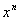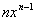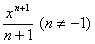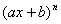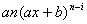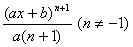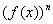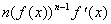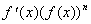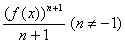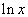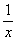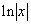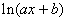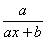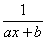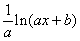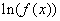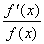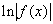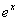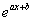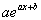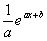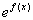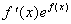Note: Calculus involving trig functions assumes you are working in RADIANS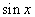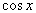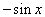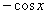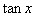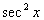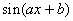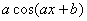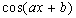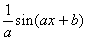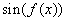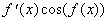similarly for cos and tan similarly for sin and sec2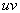Product Rule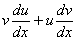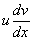Integration by parts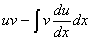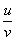Quotient Rule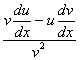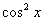Use double angle formula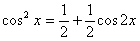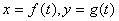Parametric differentiation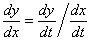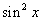Use double angle formula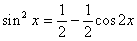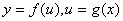Chain Rule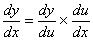Method of substitution: 1. substitute for dx in terms of du 2. substitute for x in terms of u 3. change limits to u Always look for simplifications before the next stage. Useful Relation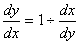General method: 1.  Is it a standard integral? 2.  Is it a reverse chain rule?(can you spot a function and its derivative?) 3. Can you split it up into two or more easier integrals? e.g.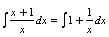. 4 Can you use partial fractions? 5. Can you use a trig formula? 6. Can you do it by parts? 7. Can you use a substitution? More than one method may work; which is the easiest?

Warning: You cannot differentiate or integrate the parts of a product or quotient separately: consider multiplying or dividing out first or using another method.